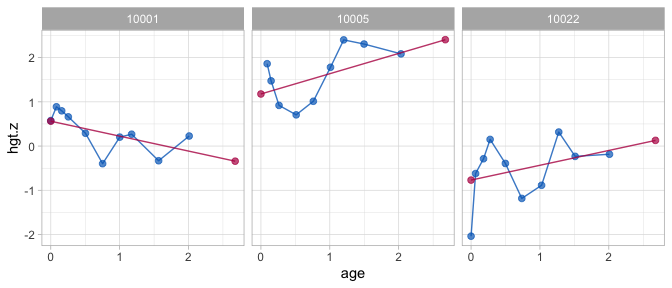# Help for old friends

#### 2020-10-23

library(brokenstick)

# Background

Versions after brokenstick 0.62.1 (May 2020) have a substantially changed interface. In particular, later versions (starting with brokenstick 0.70.0) include the following changes:

1. brokenstick adopts the tidymodels philosophy. It is now possible to fit a model using four different interfaces. There is no need anymore the hardcode variable names in the source data.

2. Version 0.70.0 introduces a new estimation method, the Kasim-Raudenbush sampler. The new method is more flexible and faster than lme4::lmer() when the number of knots is large.

3. Version 0.70.0 introduces two simple correlation models that may be used to smooth out the variance-covariance matrix of the random effects.

4. The definition of the brokenstick class has changed. Objects of class brokenstick do no longer store the training data.

5. The brokenstick_export class is retired.

6. The predict() function is fully rewritten as has now a new interface. Since the brokenstick class does not store the training data anymore, the predict() function now obtains a new_data argument. Syntax that worked for brokenstick package before 0.70.0 does not work anymore and should be updated. The shape argument replaces the output argument.

7. The plot() function is rewritten, and now requires a new_data specification.

8. Replaced functions: brokenstick() replaces fit_brokenstick(), predict.brokenstick() replaces predict.brokenstick_export(), get_r2() replaces get_pev()

9. Removed functions: get_data(), get_X(), export()

# Install legacy version

We recommend changing your code to reflect the above changes. If needed, the old version 0.62.1 can be installed as

library(devtools)
install_github("growthcharts/brokenstick@V0.62.1")

# Examples

## Example 1: Fit model

Fit model, old version:

data <- brokenstick::smocc_200
fit <- brokenstick(y = data$hgt.z, x = data$age, subjid = data\$subjid)

Fit model, version 0.75.0 and higher:

data <- brokenstick::smocc_200

# formula interface
fit1 <- brokenstick(hgt.z ~ age | id, data)

# XY interface - numeric vector
fit2 <- with(data, brokenstick(age, hgt.z, id))

# XY interface - data.frame
fit3 <- with(data, brokenstick(data.frame(age), hgt.z, id))

# XY interface - matrix
tt <- as.matrix(data[, c(1, 2, 7)])
fit4 <- brokenstick(tt[, "age", drop = FALSE],
tt[, "hgt.z", drop = FALSE],
tt[, "id", drop = FALSE])

All fitted models are identical.

## Example 2: Predict model

Predict model, old version:

# predict at observed data
p1 <- predict(fit)

# predict at knots
p2 <- predict(fit, at = "knots")

# predict at both observed data and knots
p3 <- predict(fit, at = "both")

p4 <- predict(fit, at = "knots", output = "broad")

Predict model, new version:

# predict at observed data
p1 <- predict(fit1, data)

# predict at knots
p2 <- predict(fit1, data, x = "knots")

# predict at both observed data and knots
p3 <- predict(fit1, data, x = "knots", strip_data = FALSE)

p4 <- predict(fit1, data, x = "knots", shape = "wide")

## Example 3: Plot model

Plot trajectories, old version

ids <- c(10001, 10005, 10022)
plot(fit, ids = ids)

Plot trajectories, new version

ids <- c(10001, 10005, 10022)
plot(fit1, data, group = ids, what = "all")## Example 4: Explained variance

Proportion explained variance, old version

get_pev(fit)
get_r2(fit1, data)
#>  0.81

• Main functions
• Plot trajectories
• Orginal scale and $$Z$$-score scale
• 1-line model
• 2-line broken stick model
• 9-line broken stick model
• Prediction
• Subject-level analysis
• Broken Stick Model for Irregular Longitudinal Data
• Irregular observation times
• Literature overview
• Definition of the model
• Interpretation of the model
• Estimation by lmer and kr methods
• Software overview
• brokenstick() for model fitting
• predict() for trajectory plotting
• Conversion back and forth to the $$Z$$-score scale
• Predict growth curve of new subjects
• Assess the quality of the model
• Knot placement strategies
• Critical periods
• Time-to-time correlations
• Profile analysis
• Curve interpolation
• Multiple imputation
• Curve matching
• Discussion
• Perfect model
• Properties of the perfect model
• Estimating time-to-time correlations